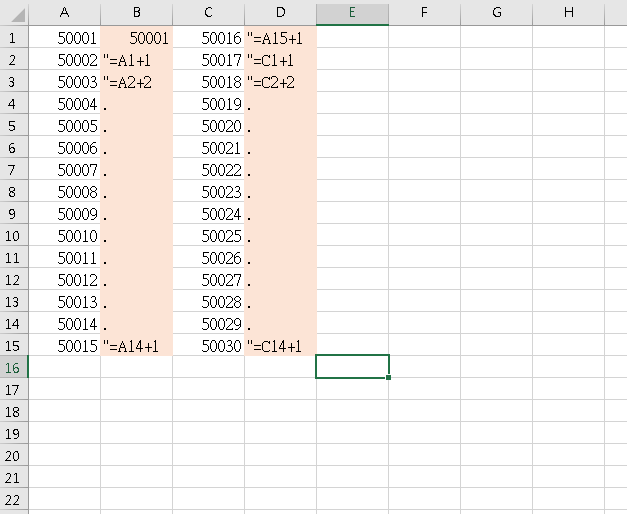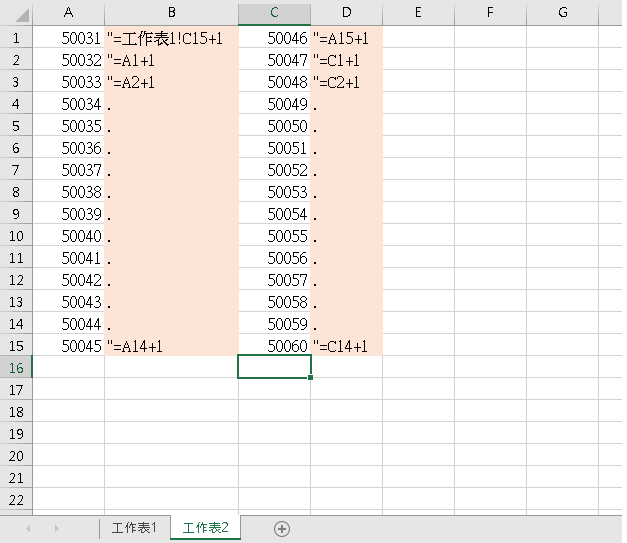#0

## Excel問題_跨分頁_流水號(在工作表三，就"=工作表2!C15+1

### 1 個回答

0

``````Sub itHelp()
Dim newName as string, i as integer

for i = 1 to worksheets.count
newName = worksheets(i).range("a1").value
newName = newName & "-" & worksheets(i).range("C15").value
worksheets(i).name = newName

if i > 1 then
worksheets(i).range("A1").formula= "='" & worksheets(i-1).name & "'!C15+1"
end if
next i
end sub
``````